Courses

# RD Sharma Solutions: Number System- 2 Class 9 Notes | EduRev

## Class 9 : RD Sharma Solutions: Number System- 2 Class 9 Notes | EduRev

The document RD Sharma Solutions: Number System- 2 Class 9 Notes | EduRev is a part of the Class 9 Course Mathematics (Maths) Class 9.
All you need of Class 9 at this link: Class 9

RD Sharma Solutions: Exercise 1.2 - Number System

Q.1. Express the following rational numbers as decimals:

(i)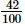(ii)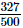(iii)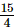Proof: (i) Given rational number is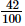Now we have to express this rational number into decimal form. So we will use long division method as below.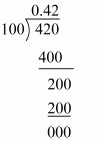Hence,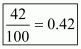(ii) Given rational number is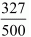Now we have to express this rational number into decimal form. So we will use long division method as below.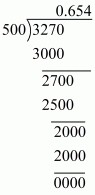Hence,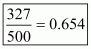(iii) Given rational number is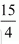Now we have to express this rational number into decimal form. So we will use long division method as below.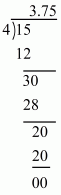Hence,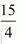= 3.75

Q.2. Express the following rational numbers as decimals:

(i)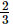(ii)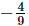(iii)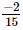(iv)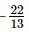(v)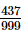(vi)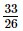Proof: (i) Given rational number is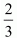Now we have to express this rational number into decimal form. So we will use long division method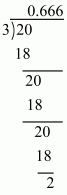Therefore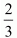= 0.6666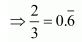Hence,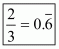(ii) Given rational number is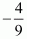Now we have to express this rational number into decimal form. So we will use long division method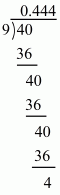Therefore,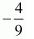= 0.444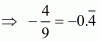Hence,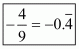(iii) Given rational number is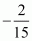Now we have to express this rational number into decimal form. So we will use long division method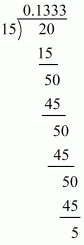Therefore= 0.1333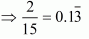Hence,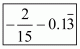(iv) Given rational number is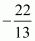Now we have to express this rational number into decimal form. So we will use long division method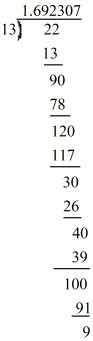Therefore=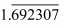Hence,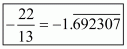(v) Given rational number is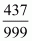Now we have to express this rational number into decimal form. So we will use long division method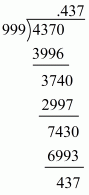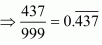Hence,(vi) Given rational number is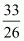Now we have to express this rational number into decimal form. So we will use long division method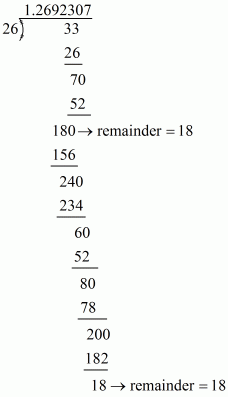Therefor=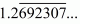Hence,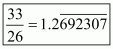Q.3. Look at several examples of rational numbers in the form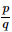(q ≠0), where p and q are integers with no common factors other than 1 and having terminating decimal representations. Can you guess what property q must satisfy?

Proof: Prime factorization is the process of finding which prime numbers you need to multiply together to get a certain number. So prime factorization of denominators (q) must have only the power of 2 or 5 or both.

Offer running on EduRev: Apply code STAYHOME200 to get INR 200 off on our premium plan EduRev Infinity!

## Mathematics (Maths) Class 9

46 videos|323 docs|108 tests

,

,

,

,

,

,

,

,

,

,

,

,

,

,

,

,

,

,

,

,

,

;